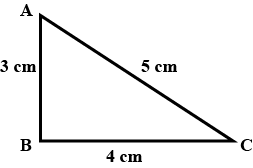# Define displacement and velocity?

Displacement is the shortest distance between two points.

For example, In the figure given below, if a person traveled from A to C through B then the displacement is the length between A and C, which is 5 units.Velocity is defined as the rate of change of displacement with respect to time. It is nothing but the speed at which an object moves in a particular direction. It is a vector quantity. It needs both magnitude and direction to represent it.

The unit of velocity is meter/second (m/s).

Mathematically, velocity is given as:

$V=\frac{D}{T}$

where, V = velocity, D = displacement, and t = time

Updated on: 10-Oct-2022

43 Views